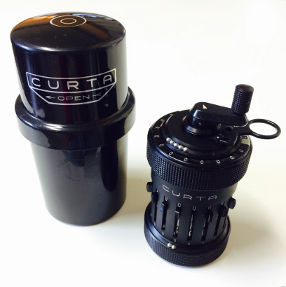# Interests

## Mechanical Calculators

I collect (hand-held) mechanical calculators. I am aways looking for weird and interesting devices. Here are some calculators in my collectionCurta
(see the picture on the left) a hand held mechanical calculator which can add, subtract, multiply, divide and compute square roots (1948 - 1970s).
Consul, the Educated Monkey
a mechanical calculator in the form of a monkey, constructed of enameled sheet metal, around 1916.
Correntator
a slide adder from the 1920s.

This is an incomplete list of {technical, fantasy} books I highly recommend to anyone iterested in cryptology, applied mathematics or fantasy.

### Cryptography, Mathematics and Computer Science

Books every computer scientist or applied mathematician should have on its bookshelf include

## Programming & Tips

I enjoy learning about programming gems and tricks to compute things efficiently or in a different way (mainly for the C-programming language).

### Bit Twiddling Hacks

A comprehensive summary of tricks to compute various basic computer operations on single computer word integers can be found at the

### Duff’s device

Tom Duff attempted to optimize the following routine, abstracted from code which copies an array of shorts into the Programmed IO data register of an Evans & Sutherland Picture System II:

send(to, from, count)
register short *to, *from;
register count;
do {
*to = *from++;
} while(--count>0);


His idea was to unwind this loop but he had to deal with the partial leftover loop. He came up with the following routine which nicely abuses the C-programming language:

send(to, from, count)
register short *to, *from;
register count;
register n=(count+7)/8;
switch(count%8) {
case 0: do {   *to = *from++;
case 7:        *to = *from++;
case 6:        *to = *from++;
case 5:        *to = *from++;
case 4:        *to = *from++;
case 3:        *to = *from++;
case 2:        *to = *from++;
case 1:        *to = *from++;
} while(--n>0);
}


## Modular inversion

The following function computes modular inversion (a−1 mod modulus) for a prime modulus and a>1 (which both fit into a standard int datatype) very efficiently. This function is from this thread on the Mersenne forum.

int single_modinv (int a, int modulus) {
int ps1, ps2, parity, dividend, divisor, rem, q, t;
q = 1;
rem = a;
dividend = modulus;
divisor = a;
ps1 = 1;
ps2 = 0;
parity = 0;
while (divisor > 1) {
rem = dividend - divisor;
t = rem - divisor;
if (t >= 0) {
q += ps1;
rem = t;
t -= divisor;
if (t >= 0) {
q += ps1;
rem = t;
t -= divisor;
if (t >= 0) {
q += ps1;
rem = t;
t -= divisor;
if (t >= 0) {
q += ps1;
rem = t;
t -= divisor;
if (t >= 0) {
q += ps1;
rem = t;
t -= divisor;
if (t >= 0) {
q += ps1;
rem = t;
t -= divisor;
if (t >= 0) {
q += ps1;
rem = t;
t -= divisor;
if (t >= 0) {
q += ps1;
rem = t;
if (rem >= divisor) {
q = dividend / divisor;
rem = dividend % divisor;
q *= ps1;
}}}}}}}}}
q += ps2;
parity = ~parity;
dividend = divisor;
divisor = rem;
ps2 = ps1;
ps1 = q;
}
if (parity == 0)
return (ps1);
else
return (modulus - ps1);
}